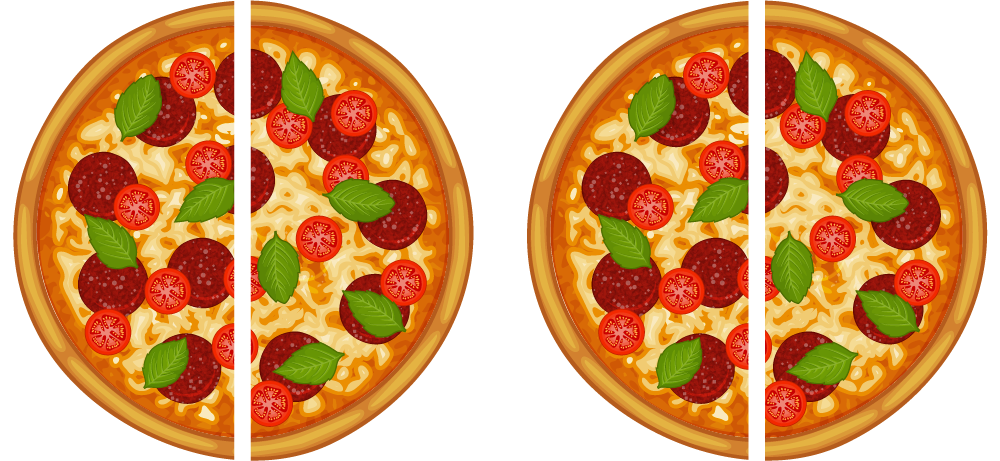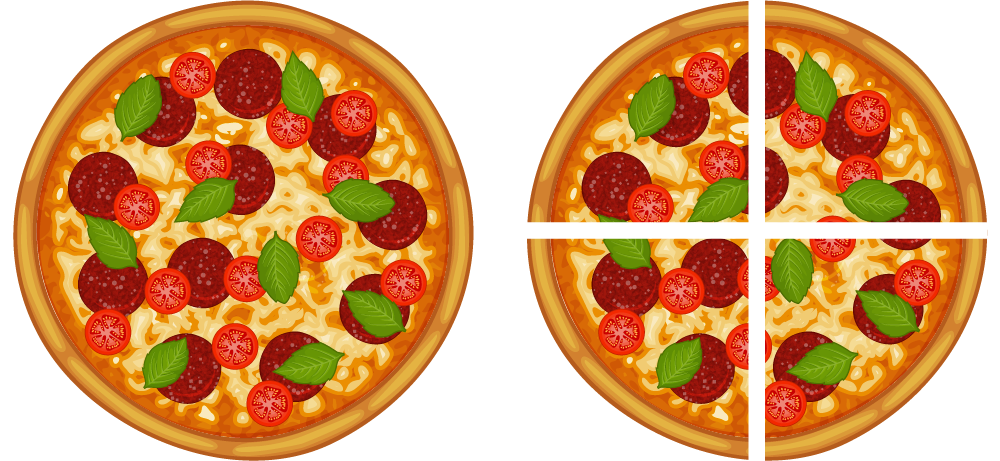Math Topics

# What Is the Value of a Fraction?

Video Crash Courses

Want to watch animated videos and solve interactive exercises about fractions? Click here to try Video Crash Courses!

Here we will take a closer look at fractions.

You are sharing two pizzas with three friends, making you four people in total. We write that as $\frac{2}{4}$. Just like the example in the previous article about fractions, you get half a pizza each. How can that be? It must mean that

 $\frac{2}{4}=\frac{1}{2}=0.5.$

You have twice as many people, but you also have twice as much pizza. For that reason, no one had to give up any pizza, and each of you were left with the same amount of pizza as before.

This shows you that two different-looking fractions might actually be equal! Maybe it seems a bit strange that a number can be written in several different ways, but it’s not any stranger than the fact that you can write your own name in both cursive writing and with capitalized letters.Looking at this picture, you can see that sharing two pizzas between four people gives each person the same amount of pizza as sharing one pizza between two people does. In both cases, each person receives half a pizza. Below, you are going to look at more fractions that can be written in several different ways, even though they are the same number. These fractions are called equivalent fractions.You and your friend are alone again, and you have one pizza to share. Last time, you sliced the pizza into two pieces and got one piece each. This time, you decide to slice the pizza into smaller pieces, and cut it into four.Each slice of pizza is now a quarter of a pizza (see above):

 $\frac{1}{4}=0.25$

As there are four slices and both of you eat the same amount, each of you get two of the four slices of pizza, $\frac{2}{4}$. In total, you ate two quarters of a pizza, which adds up to one half of a pizza—the same as last time, just with smaller slices.

Math Vault

Want to solve exercises about fractions? Try Math Vault!